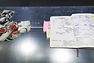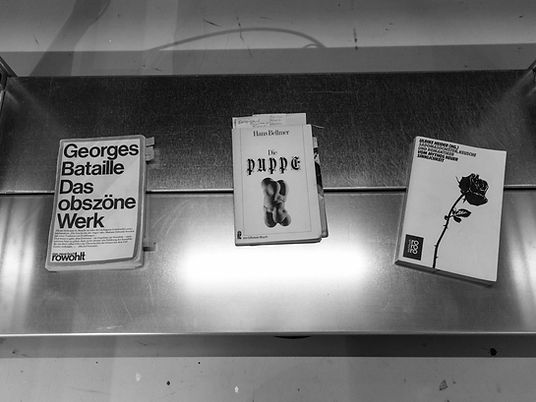f (G (sub)) = [SUB] ∞ SUB(u)

INSTALLATION

documentation, found objects, research and working materials, research results, four videos on tablets, laptop and smartphone, Errex shelves, vinyl sticker, light

f (G (sub)) = [SUB] ∞ SUB(u) examines transgression or border crossing as the French theorist, and writer Georges Bataille described and practised it. For him, transgression bore the potential of a temporary escape from the isolated separateness of the individual. In the very moment of crossing a border, he stated, a more reliable empathic communication is possible.f (G (sub)) = [SUB] ∞ SUB(u), installation view at ZHdK, Toni Areal, Zürich, CH.

Experimental series were designed and implemented to fathom and understand these secret channels. Finally, the formula for transgression was set up, and the working materials and research results were assembled in an installation.

f (G (sub)) = [SUB] ∞ SUB(u) showed at MFA Degree Show, ZHdK, Zurich, 2017.

Photos: Philip Frowein, Stefan Jaeggi

Thanks to Ian Wooldridge and Judith Welter.f (G (sub)) = [SUB] ∞ SUB(u), research material.f (G (sub)) = [SUB] ∞ SUB(u), found object and publication.f (G (sub)) = [SUB] ∞ SUB(u), photo documentation and notes.f (G (sub)) = [SUB] ∞ SUB(u), experiment documentation, tape and video.

f (G (sub)) = [SUB] ∞ SUB(u), lekker voor vrouwen, found object.f (G (sub)) = [SUB] ∞ SUB(u), research and working materials.

Natura Operans, finissage, impression.f (G (sub)) = [SUB] ∞ SUB(u), installation view at ZHdK, Toni Areal, Zürich, CH.f (G (sub)) = [SUB] ∞ SUB(u), found images, research and working material.f (G (sub)) = [SUB] ∞ SUB(u), found images, research and working material.f (G (sub)) = [SUB] ∞ SUB(u), diagram function of transgression.f (G (sub)) = [SUB] ∞ SUB(u), installation view at ZHdK, Toni Areal, Zürich, CH.f (G (sub)) = [SUB] ∞ SUB(u), working material.